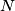### IMO Shortlist 1987 problem 21

Kvaliteta:
Avg: 0,0
Težina:
Avg: 0,0
In an acute-angled triangle$ABC$ the interior bisector of angle$A$ meets$BC$ at$L$ and meets the circumcircle of$ABC$ again at$N$. From$L$ perpendiculars are drawn to$AB$ and$AC$, with feet$K$ and$M$ respectively. Prove that the quadrilateral$AKNM$ and the triangle$ABC$ have equal areas.(IMO Problem 2)

Proposed by Soviet Union.
Izvor: Međunarodna matematička olimpijada, shortlist 1987